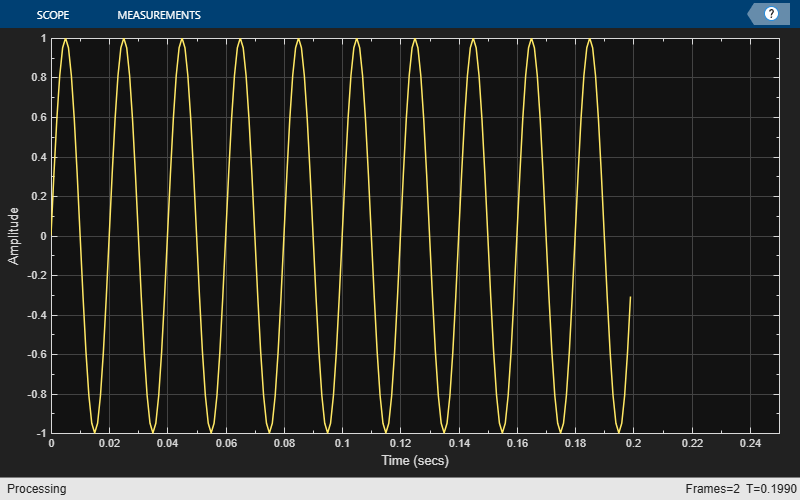# isVisible

Determine visibility of scope

## Syntax

``visibility = isVisible(scope)``

## Description

example

````visibility = isVisible(scope)` returns the visibility of the scope as logical, with 1 (true) for visible.```

## Examples

collapse all

Create a sine wave signal and view it in the scope.

```Fs = 1000; % Sample rate signal = dsp.SineWave(Frequency=50,SampleRate=Fs,... SamplesPerFrame=100); scope = timescope(SampleRate=Fs,TimeSpanSource="property",... TimeSpan=0.25,YLimits=[-1 1]); for ii = 1:2 xsine = signal(); scope(xsine) end```Hide the scope window.

```if(isVisible(scope)) hide(scope) end```

Show the scope window.

```if(~isVisible(scope)) show(scope) end```Clean up workspace variables.

`clear scope Fs sine ii xsine`

## Input Arguments

collapse all

Scope object, specified as one of the following:

• `spectrumAnalyzer` object

• `dsp.ArrayPlot` object

• `dsp.LogicAnalyzer` System object™

• `timescope` object

Example: ```myScope = spectrumAnalyzer; visibility = isVisible(myScope)```

Example: ```myTS = timescope; visibility = isVisible(myTS)```

## Output Arguments

collapse all

Scope visibility, returned as a logical `1` or a logical `0`. If the scope display is showing, the `isVisible` function returns `1` (true). Otherwise, the function returns `0` (false).

## Version History

Introduced in R2016b

expand all

Not recommended starting in R2022a